• I don't think this statement is true, nor the consequence immediately obvious given it (otherwise we could apply the same sort of argument to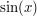for instance).

Parameterizing the arc as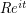for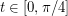, the integral over the arc is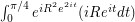.

Expanding with Euler's Formula gives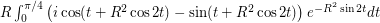.

Both the real and imaginary integrands are bounded above in absolute value by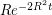, which means the integrals are bounded above by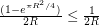and so the contribution from the arc does vanish at infinity.

• Dimension arguments in combinatorics

Why do we need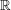instead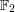in the proof of Sauer-Shelah lemma? It looks that any field would work.

• Bijections and counting

The image of each X_i ought to be {-1, 1}, I think.

• I am not quite sure where to put this article. There is a 'use convexity' link in the 'estimating integrals' section but also one for Hölder's inequality.
I guess the best would be a link from both articles (if they exist one day) to this one?

• Is there an analogue of this trick for the multidimensional Fourier transform?

• 7 years 36 weeks ago

Angela, I think you missed some coefficients, correct expression should be,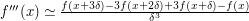And I'm sorry but its really difficult to understand the formula you write for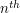derivative. I think the expression you wanted to write is the following-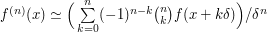• 8 years 8 weeks ago Just-do-it proofs

"It's pretty obvious that you can't cover an open disc with finitely many points and lines, but it is not quite trivial, so here's an easy proof: ..."

A simpler proof: it is impossible to cover even a circle with finitely many lines, since a line can intersect a circle at at most 2 points!

• 8 years 25 weeks ago Extra logarithmic factors

Only if squares are of the same size, no? It would also work for rectangles with same sizes, right?

• 8 years 25 weeks ago Extra logarithmic factors

In the example proof, instead of squares, isn't it meant to be rectangles equal to each other? If squares are allowed to have different sizes, I don't think it is true that each neighbour of a square must overlap with one of its vertices.

A question: is there some reference for this problem and proof? I realize this problem asks for bounds on m in terms of n, where n=R(m,m) for intersection graph of rectangles. Was this studied for other special graphs?

• 8 years 35 weeks ago The tensor power trick

1. (Approximate counting of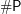problems in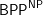)

Let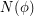be the number of satisfying assignments of a Boolean formula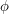. Using the leftover hash lemma, there exists a polynomial time probabilistic algorithm taking oracle for SAT that given a formulaoutputs with high probability a numbersuch that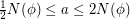i.e. approximates within factor of 2.

By replacingwith "tensor power"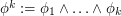, the number of assignments rises to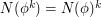. Running the algorithm on this formula,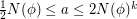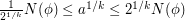and putting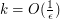we get approximation within factor ofin time polynomial inand length of. The algorithm is by Stockmeyer.

2. (Independent set cannot be approximated to a constant factor)

For any constant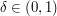,-approximating maximal independent set in a graph is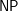-hard. (Taking complement, the same argument goes to clique)

Proof idea:

The PCP theorem implies that there is a constant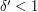such that MAX-3SAT cannot be approximated within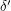. The usual reduction from 3SAT to independent set takes a formula withclauses and gives a graph with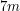vertices, where each vertex corresponds to one of 7 ways a clause can be satisfied, and edges correspond to conflicts. Therefore the approximation ratio is preserved, and independent set cannot be approximated within some constant factor. Applying "tensor square"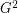allows to lower the constant arbitrarily.

• 9 years 10 weeks ago

Use any value δ, which can be made small enough to approximate the derivitive to an arbitrary number of decimal places.
f'(x)≃(f(x+δ)-f(x))/δ
f''(x) ((f(x+2δ)-f(x+δ))-(f(x+δ)-f(x)))/δ=f(x+2δ)-2f(x+δ)+f(x)
f'''(x) (f(x+3δ)-f(x+2δ)+f(x+δ)-f(x))/δ
The pattern continues. The nth derivitive is
....................n
...................⎲
f(x+nδ)/δ+ ⎳ n(-1)^k f(x+kδ)/δ
...................k=1

The dots are there because the computer won't show spaces properly.

• 9 years 10 weeks ago The second-moment method

.. but I can't understand why "the proportion of x such that | f(x) - delta N | \geq (delta N / 2)" has delta N instead of delta^2 N which is the average value of f(x) as stated previously. And I haven't been able to turn inline commenting on in google chrome, my apologies.

• 9 years 32 weeks ago Create an epsilon of room

A few other articles had also been mysteriously truncated, but I have now restored them (and this one). If anyone spots another page that does not look right then please let us know, for example via the forums.

• 9 years 33 weeks ago Create an epsilon of room

For some strange reason, all revisions of this article have been truncated at the first paragraph, and I cannot find a way to retrieve any subsequent portion of the text. Has this also occurred for other articles? Is there any way to recover some copy of the full article?

• 9 years 49 weeks ago Prove a consequence first

That's a great explanation. Thanks.

• 9 years 50 weeks ago Prove a consequence first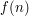is independent ofif the value ofdoes not actually depend on, i.e. it is constant as a function of. For instanceis independent of, as it is always.

Equivalently,is independent ofif one has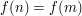for all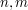.

• 9 years 50 weeks ago Prove a consequence first

Proving the consequence first is a great way to simplify a problem.
But I don't understand the example. What exactly does "independent" mean. How can f(n) be independent of n?

Sorry if this is a stupid question, but I would really like to understand this.

• 10 years 1 week ago

Suppose first that X is semialgebraic. Consider the function f :C—> R defined by

f(z)= dist(z, X)

Then for all but finitely many positive numbers t the level set C_t={ f=t} is a smooth curve. For t sufficiently small C_t will be a curve that contains X in its interior {f< t}. As an aside, for t small, the set {f\leq t} is homotopy equivalent to X.

If X is not semialgebraic, then the pixellation Y that you constructed is compact, semialgebraic, contains X and is contained in D if the size of the squares is small enough. Now apply the previous construction to Y.

PS My PhD student has been investigating approximations of planar sets by grids of squares (or pixellations). He has obtained quite interesting results. If P_r if the pixellation of a semialgebraic set with squares of size r then one can algorithmically associate to P_r a PL set S_r that in a rather strong sense to X as r–>0. In particular, many geometric invariants of S_r (such as area, perimeter, curvature measures, Betti numbers) converge to the corresponding invariants of X.

• 10 years 3 weeks ago

Isn't that always the case?

• 10 years 9 weeks ago How to use fixed point theorems

There are good reasons to study not just the fixed point property (fpp) but the common generalization of fpp and the covering dimension theory, namely the universal functions, and even the universal (and couniversal) morphisms for general categories. (Since monoids are 1-object categories, one may also focus on the universal elements of monoids).

• 10 years 9 weeks ago Functional analysis front page

Hi,

First of all, an excellent site! It's great to have such a compendium of mathematical knowledge online, accessable to all. So a great deal of thanks goes to the creators of this site!

I'm in Year 12 in Australia and I just stumbled upon this page [on functional analysis], and I'd love to see it expanded. It looks to be an interesting field! I assume that capital Omega here is a space/topology, maybe even a set being integrated over? Could an explanation be given of the theory behind L^p(Omega) spaces and how it ties in more broadly to this topic?

I look forward to hearing further (and just as a side-note, I believe my mathematical background to be good so far - I have a good understanding of convergence of sequences, Maclaurin series, complex numbers, derivatives of inverse trigonometric functions, etc). I have read a little of analysis, to which I have been impressed so far, but that I have only broadly skimmed.

Thanks once again.
Davin

• 10 years 11 weeks ago

I am sorry. I know that the definition is not *wrong* with a correct definition of set theory (which is usually not given in the books that use the above definition of ordered pair), so I reverted it. I just think that it is crazy to unnecessarily use that as definition, but it seems that many people love it.

• 10 years 17 weeks ago

The use of this method for Zeta(3) is more involved but goes deeper into the principles of this method. I suggest that someone try to write up a description of this as Example 3.

• 10 years 17 weeks ago

This is indeed one instance of one of the most general method useful in mathematics and all the sciences. Z. A. Melzak has devoted a whole book to this idea : "Bypasses" (Wiley, 1983) showing how it encompasses geometry, algebra, analysis, physics, chemistry, information theory, technology in general.

In this tricki entry, contributors have shown classical instances of how to find and build T (a transformation toward another form) motivated by the fact we know how to do U (the transformation/projection of the target form into needed information, here its solutions), mainly with algebraic objects.

One of the most important aspect of this method or trick is that it can be nested at will.

• 10 years 27 weeks ago

e+e^(-1) has sequence of approximations 2(1 + 1/2! + 1/4! + ... 1/(2n)!) and no immediately obvious (to me) formula for the continued-fraction expansion.